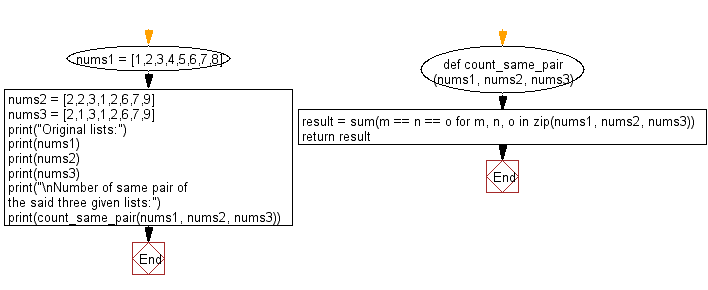﻿ Python: Count the same pair in three given lists - w3resource# Python: Count the same pair in three given lists

## Python List: Exercise - 130 with Solution

Write a Python program to count the same pair in three given lists.

Sample Solution:

Python Code:

``````def count_same_pair(nums1, nums2, nums3):
result = sum(m == n == o for m, n, o in zip(nums1, nums2, nums3))
return result
nums1 = [1,2,3,4,5,6,7,8]
nums2 = [2,2,3,1,2,6,7,9]
nums3 = [2,1,3,1,2,6,7,9]
print("Original lists:")
print(nums1)
print(nums2)
print(nums3)
print("\nNumber of same pair of the said three given lists:")
print(count_same_pair(nums1, nums2, nums3))
```
```

Sample Output:

```Original lists:
[1, 2, 3, 4, 5, 6, 7, 8]
[2, 2, 3, 1, 2, 6, 7, 9]
[2, 1, 3, 1, 2, 6, 7, 9]

Number of same pair of the said three given lists:
3
```

Flowchart:## Visualize Python code execution:

The following tool visualize what the computer is doing step-by-step as it executes the said program:

Python Code Editor:

Have another way to solve this solution? Contribute your code (and comments) through Disqus.

What is the difficulty level of this exercise?

Test your Python skills with w3resource's quiz

﻿

## Python: Tips of the Day

```print(2_000_000)
```2000000